The MCAT CARS Strategy Course begins February 27!

### Electrostatics

Written by Ryan on Jan 5, 2020

## Electric Charge in the Atom

Atoms contain negatively charged electrons and positively charged protons; the number of each determines the atom’s net charge.

### LEARNING OBJECTIVES

Identify factors that determine the atom’s net charge

### KEY TAKEAWAYS

#### Key Points

• A proton is a positively charged particle located in the nucleus of an atom. An electron has
$\frac{1}{1836}$

times the mass of a proton, but an equal and opposite negative charge.

• An elementary charge — that of a proton or electron — is approximately equal to 1.6×10-19Coulombs.
• Unlike protons, electrons can move from atom to atom. If an atom has an equal number of protons and electrons, its net charge is 0. If it gains an extra electron, it becomes negatively charged and is known as an anion. If it loses an electron, it becomes positively charged and is known as a cation.

#### Key Terms

• nucleus: the massive, positively charged central part of an atom, made up of protons and neutrons

### Overview of Atomic Electrical Charges

Atoms, the fundamental building blocks of all molecules, consist of three types of particles: protons, neutrons, and electrons. Of these three subatomic particle types, two (protons and electrons) carry a net electric charge, while neutrons are neutral and have no net charge.

Both protons and electrons have charge that is quantized. That is, the magnitude of their respective charges, which are equal each other, is 1. This standard value is equal to approximately 1.6×10-19 Coulombs.

### Protons

Protons are found in the center of the atom; they, with neutrons, make up the nucleus. Protons have a charge of +1 and a mass of 1 atomic mass unit, which is approximately equal to 1.66×10-24 grams. The number of protons in an atom defines the identity of the element (an atom with 1 proton is hydrogen, for example, and an atom with two protons is helium). As such, protons are relatively stable; their number rarely changes, only in the instance of radioactive decay.

### Electrons

Electrons are found in the periphery of the atom and have a charge of -1. They are much smaller than protons; their mass is

$\frac{1}{1836}$

amu. Typically in modeling atoms, protons and neutrons are regarded as stationary, while electrons move about in the space outside the nucleus like a cloud. The negatively charged electronic cloud indicates the regions of the space where electrons are likely to be found. The electrons cloud patterns are extremely complex and is of no importance to the discussion of electric charge in the atom. More important is the fact that electrons are labile; that is, they can be transferred from one atom to the next. It is through electronic transfer that atoms become charged.

### Ions

In the ground state, an atom will have an equal number of protons and electrons, and thus will have a net charge of 0. However, because electrons can be transferred from one atom to another, it is possible for atoms to become charged. Atoms in such a state are known as ions.

If a neutral atom gains an electron, it becomes negative. This kind of ion is called an anion.

If a neutral atom loses an electron, it becomes positive. This kind of ion is called a cation.

The steady flow of electrons is called current. Current is what flows through electrical wires and powers electronics items, from light bulbs to televisions.

Electric Charge: A brief overview of atoms, ions, and electrical charge.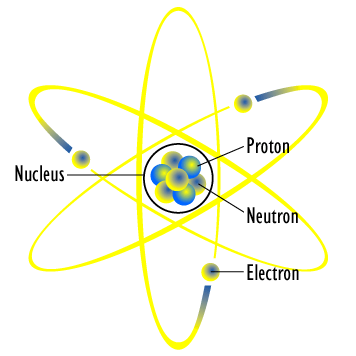Planetary Model of an Atom: Small electrons orbit the large and relatively fixed nucleus of protons and neutrons.

## Properties of Electric Charges

Electric charge is a fundamental physical property of matter that has many parallels to mass.

### LEARNING OBJECTIVES

Describe properties of electric charge, such as its relativistic invariance and its conservation in closed systems

### KEY TAKEAWAYS

#### Key Points

• Charge is measured in Coulombs (C), which represent 6.242×1018 e, where e is the charge of a proton. Charges can be positive or negative, and as such a singular proton has a charge of 1.602×10−19 C, while an electron has a charge of -1.602×10−19 C.
• Electric charge, like mass, is conserved. The force generated by two charges is of the same form as that generated by two masses and, like gravity, force from an electrical field is both conservative and central.
• Electric charge is a relativistic invariant. That is, charge (unlike mass) is independent of speed. Whereas the mass of a particle will exponentially rise as its speed approaches that of light, charge will remain constant.

#### Key Terms

• coulomb: In the International System of Units, the derived unit of electric charge; the amount of electric charge carried by a current of 1 ampere flowing for 1 second. Symbol: C
• gravity: Resultant force on Earth’s surface, of the attraction by the Earth’s masses, and the centrifugal pseudo-force caused by the Earth’s rotation.
• electric field: A region of space around a charged particle, or between two voltages; it exerts a force on charged objects in its vicinity.

### Properties of Electric Charge

Electric charge, like mass and volume, is a physical property of matter. Its SI unit is known as the Coulomb (C), which represents 6.242×1018e, where e is the charge of a proton. Charges can be positive or negative; a singular proton has a charge of 1.602×10−19 C, while an electron has a charge of -1.602×10−19 C.

### Invariance

Like mass, electric charge in a closed system is conserved. As long as a system is impermeable, the amount of charge inside it will neither increase nor decrease; it can only be transferred. However, electric charge differs from other properties—like mass—in that it is a relativistic invariant. That is, charge is independent of speed. The mass of a particle will rise exponentially as its speed approaches that of light, its charge, however, will remain constant.

The independence of electric charge from speed was proven through an experiment in which one fast-moving helium nucleus (two protons and two neutrons bound together) was proven to have the same charge as two separate, slow-moving deuterium nuclei (one proton and one neutron bound together in each nucleus).

### Attraction and Repulsion

Electric charge is a property that produces forces that can attract or repel matter. Mass is similar, although it can only attract matter, not repel it. Still, the formula describing the interactions between charges is remarkably similar to that which characterizes the interactions between masses. For electric fields, the force (F) is related to the charges (q1, q2) and the distance (r) between them as:

$\text{F}=\frac{1}{4\pi {ϵ}_{0}}\frac{{\text{q}}_{1}{\text{q}}_{2}}{{\text{r}}^{2}}$

where π and

${ϵ}_{0}$

are constants. This is known as Coulomb’s Law.Coulomb’s Law: The forces (F1 and F2) sum to produce the total force, which is calculated by Coulomb’s Law and is proportional to the product of the charges q1 and q2, and inversely proportional to the square of the distance (r21) between them.

The formula for gravitational force has exactly the same form as Coulomb’s Law, but relates the product of two masses (rather than the charges) and uses a different constant. Both act in a vacuum and are central (depend only on distance between the forces) and conservative (independent of path taken). However, it should be noted that when comparing similar terms, charge-based interaction is substantially greater than that based on mass. For example, the electric repulsion between two electrons is about 1042 times stronger than their gravitational attraction.

## Charge Separation

Charge separation, often referred to as static electricity, is the building of space between particles of opposite charges.

### LEARNING OBJECTIVES

Identify factors that can create charge separation

### KEY TAKEAWAYS

#### Key Points

• Because electrons are labile (i.e., they can be transferred from atom to atom), it is possible for “charge separation” to occur. This phenomenon is often commonly referred to as static electricity.
• Charge separation can be created by friction, pressure, heat, and other charges.
• Charge separation can reach a critical level, whereat it is discharged. Lightning is a common example.

#### Key Terms

• discharge: the act of releasing an accumulated charge
• static electricity: an electric charge that has built up on an insulated body, often due to friction
• nucleus: the massive, positively charged central part of an atom, made up of protons and neutrons

All matter is composed of atoms made up of negatively-charged electrons and positively-charged protons. In the ground state, each atom is of neutral charge—its protons and electrons are equal in number, and it exists with no permanent dipole. Because electrons are labile (i.e., they can be transferred from atom to atom) it is possible for the phenomenon of “charge separation” (often referred to as static electricity) to occur.Static Electricity: Due to friction between her hair and the plastic slide, the girl on the left has created charge separation, resulting in her hair being attracted to the slide.

In chemistry, this charge separation is illustrated simply by the transfer of an electron from one atom to another as an ionic bond is formed. In physics, there are many other instances of charge separation that cannot be written as formal chemical reactions. Consider, for example, rubbing a balloon on your hair. Once you pull the balloon away, your hair will stand on end and “reach” towards the balloon. This is because electrons from one have transferred to the other, causing one to be positive and the other to be negative. Thus, the opposite charges attract. A similar example can be seen in playground slides (as shown in ).

Charge separation can be created not only by friction, but by pressure, heat, and other charges. Both pressure and heat increase the energy of a material and can cause electrons to break free and separate from their nuclei. Charge, meanwhile, can attract electrons to or repel them from a nucleus. For example, a nearby negative charge can “push” electrons away from the nucleus around which they typically orbit. Charge separation occurs often in the natural world. It can have an extreme effect if it reaches a critical level, whereat it becomes discharged. Lightning is a common example.

## Polarization

Dielectric polarization is the phenomenon that arises when positive and negative charges in a material are separated.

### LEARNING OBJECTIVES

Identify two ways polarization can occur on the molecular level

### KEY TAKEAWAYS

#### Key Points

• Dielectrics are insulators that are capable of being polarized by an electric field. That is, their charges cannot flow freely, but can still be induced to redistribute unevenly.
• Electric fields applied to atoms will push electrons away from the field. In the case of polar molecules, the negative ends thereof will align themselves away from the field while the positive ends will be towards the field.
• An instantaneous polarization occurs when ions, through natural, random vibrations, become distributed asymmetrically such that one area is more dense with one type of ion than another.

#### Key Terms

• dipole moment: The vector product of the charge on either pole of a dipole and the distance separating them.
• dielectric: An electrically insulating or nonconducting material considered for its electric susceptibility (i.e., its property of polarization when exposed to an external electric field).
• insulator: A substance that does not transmit heat (thermal insulator), sound (acoustic insulator) or electricity (electrical insulator).

The concept of polarity is very broad and can be applied to molecules, light, and electric fields. For the purposes of this atom, we focus on its meaning in the context of what is known as dielectric polarization—the separation of charges in materials.

### Dielectrics

A dielectric is an insulator that can be polarized by an electric field, meaning that it is a material in which charge does not flow freely, but in the presence of an electric field it can shift its charge distribution. Positive charge in a dielectric will migrate towards the applied field, while negative charges will shift away. This creates a weak local field within the material that opposes the applied field.

Different materials will react differently to an induced field, depending on their dielectric constant. This constant is the degree of their polarizability (the extent to which they become polarized).

### Atomic Model

The most basic view of dielectrics involves considering their charged components: protons and electrons. If an electric field is applied to an atom, the electrons in the atom will migrate away from the applied field. The protons, however, remain relatively exposed to the field. This separation creates a dipole moment, as shown in.Reaction of an Atom to an Applied Electric Field: When an electric field (E) is applied, electrons drift away from the field. Their average location is displaced from the average location of the protons (which hasn’t moved) by a distance of d. The atom’s dipole moment is represented by M.

### Dipole Polarization

On the molecular level, polarization can occur with both dipoles and ions. In polar bonds, electrons are more attracted to one nucleus than to the other. One example of a dipole molecule is water, (H2O), which has a bent shape (the H-O-H angle is 104.45°) and in which the oxygen pulls electron density away from the H atoms, leaving the H relatively positive and the O relatively negative, as shown in.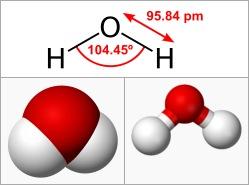Water Molecule: Water is an example of a dipole molecule, which has a bent shape (the H-O-H angle is 104.45°) and in which the oxygen pulls electron density away from the H atoms, leaving the H relatively positive and the O relatively negative.

When a dipolar molecule is exposed to an electric field, the molecule will align itself with the field, with the positive end towards the electric field and the negative end away from it.

### Ionic Polarization

Ionic compounds are those that are formed from permanently charge-separated ions. For example, table salt (NaCl) is formed from Na+ and Cl– ions that are not formally bound to one another through a chemical bond, but interact very strongly due to their opposite charges.

Ions are still free from one another and will naturally move at random. If they happen to move in a way that is asymmetrical, and results in a greater concentration of positive ions in one area and a greater concentration of negative ions in another, the sample of ionic compound will be polarized—a phenomenon is known as ionic polarization.

## Static Electricity, Charge, and the Conservation of Charge

Electric charge is a physical property that is perpetually conserved in amount; it can build up in matter, which creates static electricity.

### LEARNING OBJECTIVES

Formulate rules that apply to the creation and the destruction of electric charge

### KEY TAKEAWAYS

#### Key Points

• Electric charge is a physical property of matter created by an imbalance in the number of protons and electrons in a substance.
• Charge can be created or destroyed. However, any creation or elimination of charge occurs at a ratio of 1:1 between positive and negative charges.
• Static electricity is when an excess of electric charge collects on an object’s surface.

#### Key Terms

• electric charge: A quantum number that determines the electromagnetic interactions of some subatomic particles; by convention, the electron has an electric charge of -1 and the proton +1, and quarks have fractional charge.
• discharge: the act of releasing an accumulated charge
• static electricity: an electric charge that has built up on an insulated body, often due to friction

Electric charge is a physical property of matter. It is created by an imbalance in a substance’s number of protons and electrons. The matter is positively charged if it contains more protons than electrons, and it is negatively charged if it contains more electrons than protons. In both instances, charged particles will experience a force when in the presence of other charged matter.

Charges of like sign (positive and positive, or negative and negative) will repel each other, whereas charges of opposite sign (positive and negative) will attract each another, as shown in.Charge Repulsion and Attraction: Charges of like sign (positive and positive, or negative and negative) will repel each other, whereas charges of opposite sign (positive and negative) will attract each other.

The SI unit for charge is the Coulomb (C), which is approximately equal to

$6.24×{10}^{18}$

elementary charges. (An elementary charge is the magnitude of charge of a proton or electron. )

### Conservation of Charge

Charge, like matter, is essentially constant throughout the universe and over time. In physics, charge conservation is the principle that electric charge can neither be created nor destroyed. The net quantity of electric charge, the amount of positive charge minus the amount of negative charge in the universe, is always conserved.

For any finite volume, the law of conservation of charge (Q) can be written as a continuity equation:

$\text{Q}\left({\text{t}}_{2}\right)=\text{Q}\left({\text{t}}_{1}\right)+{\text{Q}}_{\text{in}}-{\text{Q}}_{\text{out}}$

where Q(t1) is the charge in the system at a given time, Q(t2) is the charge in the same system at a later time, Qin is the charge that has entered the system between the two times, and Qout is the amount of charge that has left the system between the two times.

This does not mean that individual positive and negative charges cannot be created or destroyed. Electric charge is carried by subatomic particles such as electrons and protons, which can be created and destroyed. For example, when particles are destroyed, equal numbers of positive and negative charges are destroyed, keeping the net amount of charge unchanged.

### Static Electricity

Static electricity is when an excess of electric charge collects on an object’s surface. It can be created through contact between materials, a buildup of pressure or heat, or the presence of a charge. Static electricity can also be created through friction between a balloon (or another object) and human hair (see ). It can be observed in storm clouds as a result of pressure buildup; lightning (see ) is the discharge that occurs after the charge exceeds a critical concentration.Static Electricity: Due to friction between her hair and the plastic slide, the girl on the left has created charge separation, resulting in her hair being attracted to the slide.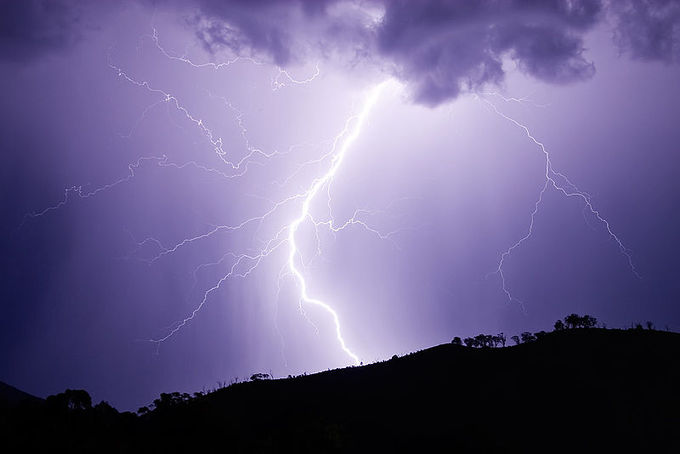Lightning: Lightning is a dramatic natural example of static discharge.

## Conductors and Insulators

Based on the ability to conduct current, materials are divided into conductors and insulators.

### LEARNING OBJECTIVES

Identify conductors and insulators among common materials

### KEY TAKEAWAYS

#### Key Points

• Resistivity, a physical property that measures the ability of a material to carry current, is the main factor in determining whether a substance is a conductor or an insulator.
• Conductors contain electric charges that, when exposed to a potential difference, move towards one pole or the other. This flow of charge is electric current.
• Insulators are materials in which the internal charge cannot flow freely, and thus cannot conduct electric current to an appreciable degree when exposed to an electric field.

#### Key Terms

• conductor: A material which contains movable electric charges.
• insulator: A substance that does not transmit heat (thermal insulator), sound (acoustic insulator) or electricity (electrical insulator).
• resistivity: In general, the resistance to electric current of a material; in particular, the degree to which a material resists the flow of electricity.

### Overview

All materials can be categorized as either insulators or conductors based on a physical property known as resistivity.

An insulator is a material in which, when exposed to an electric field, the electric charges do not flow freely—it has a high resistivity. Conversely, a conductor is a material that permits the flow of electric charges in one or more directions—its resistivity is low.

### Conductors

All conductors contain electric charges that, when exposed to a potential difference, move towards one pole or the other. The positive charges in a conductor will migrate towards the negative end of the potential difference; the negative charges in the material will move towards the positive end of the potential difference. This flow of charge is electric current.

Ionic substances and solutions can conduct electricity, but the most common and effective conductors are metals. Copper is commonly used in wires due to its high conductivity and relatively inexpensive price. However, gold-plated wires are sometimes used in instances in which especially high conductivity is necessary.

Every conductor has a limit to its ampacity, or amount of current it can carry. This usually is the current at which the heat released due to resistance melts the material.

### Insulators

Insulators are materials in which the internal charge cannot flow freely, and thus cannot conduct electric current to an appreciable degree when exposed to an electric field.

While there is no perfect insulator with infinite resistivity, materials like glass, paper and Teflon have very high resistivity and can effectively serve as insulators in most instances.

Just as conductors are used to carry electrical current through wires, insulators are commonly used as coating for the wires.

Insulators, like conductors, have their physical limits. When exposed to enough voltage, an insulator will experience what is known as electrical breakdown, in which current suddenly spikes through the material as it becomes a conductor.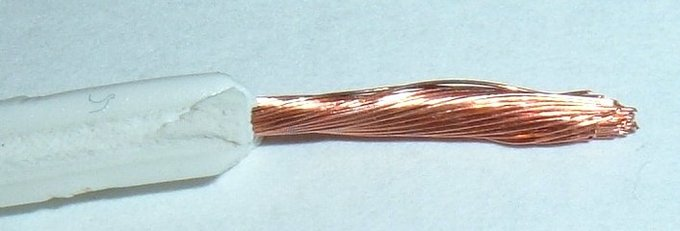Conductor and Insulator in a Wire: This wire consists of a core of copper (a conductor) and a coating of polyethylene (an insulator). The copper allows current to flow through the wire, while the polyethylene ensures that the current does not escape.

## The Millikan Oil-Drop Experiment

In 1911, using charged droplets of oil, Robert Millikan was able to determine the charge of an electron.

### LEARNING OBJECTIVES

Explain the difference in value of a real electron’s charge and the charge measured by Robert Millikan

### KEY TAKEAWAYS

#### Key Points

• The Oil-Drop Experiment involved ionizing droplets of oil as they fell through the air, and balancing the force of gravity with the force of an electric field applied by electrodes above and below the droplet.
• Millikan could not directly count the number of electrons on each oil droplet, but found that the common denominator between all measured charges was equal to 1.5924(17)×10−19 C, and thus concluded that this value was the charge of an electron.
• The measured value of an electron’s charge, 1.5924(17)×10−19 C, differs from the accepted value of 1.602176487(40)×10−19 C by less than one percent.

#### Key Terms

• voltage: The amount of electrostatic potential between two points in space.
• terminal velocity: The speed at which an object in free-fall and not in a vacuum ceases to accelerate downwards because the force of gravity is equal and opposite to the drag force acting against it.
• electric field: A region of space around a charged particle, or between two voltages; it exerts a force on charged objects in its vicinity.

### The Oil-Drop Experiment

The Oil-Drop Experiment, otherwise known as the Millikan Oil-Drop Experiment, is one of the most influential studies in the history of physical science.

Performed by Robert Millikan and Harvey Fletcher in 1911, the experiment was designed to determine the charge of a single electron, otherwise known as the elementary electric charge.

Millikan designed his experiment to measure the force on oil droplets between two electrodes.

He used an atomizer to spray a mist of tiny oil droplets into a chamber, which included a hole. Some droplets would fall through this hole and into a chamber, where he measured their terminal velocity and calculated their mass.

Millikan then exposed the droplets to X-rays, which ionized molecules in the air and caused electrons to attach to the oil droplets, thus making them charged. The top and bottom of the chamber were attached to a battery, and the potential difference between the top and bottom produced an electric field that acted on the charged oil drops.

Adjusting the voltage perfectly, Millikan was able to balance the force of gravity (which was exerted downward) with the force of the electric field on the charged particles (which was exerted upward), causing the oil droplets to be suspended in mid-air.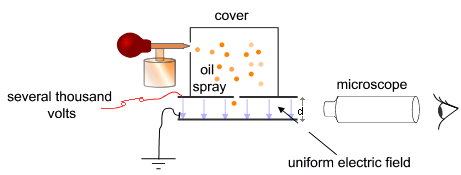Simplified scheme of Millikan’s oil-drop experiment: This apparatus has a parallel pair of horizontal metal plates. A uniform electric field is created between them. The ring has three holes for illumination and one for viewing through a microscope. Special oil for vacuum apparatus is sprayed into the chamber, where drops become electrically charged. The droplets enter the space between the plates and can be controlled by changing the voltage across the plates.

Millikan then calculated the charge on particles suspended in mid-air. His assumptions were that the force of gravity, which is the product of mass (m) and gravitational acceleration (g), was equal to the force of the electric field (the product of the charge (q) and the electric field (E)):

$\text{q}\cdot \text{E}=\text{m}\cdot \text{g}$

$\text{q}=\frac{\text{m}\cdot \text{g}}{\text{E}}$

Since he already knew the mass of the oil droplets and the acceleration due to gravity (9.81 m/s^2), as well as the energy of the x-rays he was using, he was able to calculate the charge.

Although the charge of each droplet was unknown, Millikan adjusted the strength of the X-rays ionizing the air and measured many values of (q) from many different oil droplets. In each instance, the charge measured was a multiple of 1.5924(17)×10−19 C. Thus, it was concluded that the elementary electric charge was 1.5924(17)×10−19 C.

The results were very accurate. The calculated value from the Oil-Drop Experiment differs by less than one percent of the current accepted value of 1.602176487(40)×10−19 C.

The Oil-Drop Experiment was tremendously influential at the time, not only for determining the charge of an electron, but for helping prove the existence of particles smaller than atoms. At the time, it was not fully accepted that protons, neutrons, and electrons existed.

Billing Information